An open knife edge of mass 'm' is dropped from a height 'h' on a wooden floor. If the blade penetrates upto the depth 'd' into the wood, the average resistance offered by the wood to the knife edge is

(1) mg

(2) $mg\left(1-\frac{h}{d}\right)$

(3) $mg\left(1+\frac{h}{d}\right)$

(4) $mg{\left(1+\frac{h}{d}\right)}^{2}$

Concept Questions :-

Gravitational Potential energy
High Yielding Test Series + Question Bank - NEET 2020

Difficulty Level:

A body is moving along a straight line by a machine delivering constant power. The distance moved by the body in time t is proportional to

(1) t1/2

(2) t3/4

(3) t3/2

(4) t2

Concept Questions :-

Power
High Yielding Test Series + Question Bank - NEET 2020

Difficulty Level:

A batsman hits a sixer and the ball touches the ground outside the cricket field. Which of the following graph describes the variation of the cricket ball's vertical velocity v with time between the time t1 as it hits the bat and time t2 when it touches the ground

(1)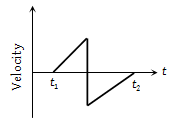(2)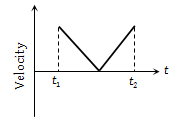(3)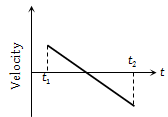(4)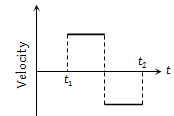Concept Questions :-

Work-Energy theorem
High Yielding Test Series + Question Bank - NEET 2020

Difficulty Level:

The relationship between force and position is shown in the figure given (in one dimensional case). The work done by the force in displacing a body from x = 1 cm to x = 5 cm is -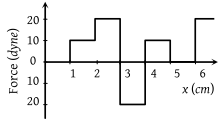(1) 20 ergs

(2) 60 ergs

(3) 70 ergs

(4) 700 ergs

Concept Questions :-

Work done by variable force
High Yielding Test Series + Question Bank - NEET 2020

Difficulty Level:

The pointer reading v/s load graph for a spring balance is as given in the figure. The spring constant is-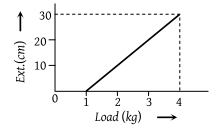(1) 0.1 N/cm

(2) 5 N/cm

(3) 0.3 N/cm

(4) 1 N/cm

Concept Questions :-

Elastic potential energy
High Yielding Test Series + Question Bank - NEET 2020

Difficulty Level:

Adjacent figure shows the force-displacement graph of a moving body, the work done in displacing body from x = 0 to x = 35 m is equal to-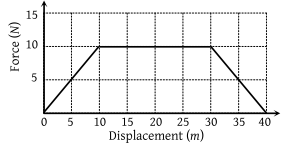(1) 50 J

(2) 25 J

(3) 287.5 J

(4) 200 J

Concept Questions :-

Work done by variable force
High Yielding Test Series + Question Bank - NEET 2020

Difficulty Level:

A 10kg mass moves along x-axis. Its acceleration as a function of its position is shown in the figure. What is the total work done on the mass by the force as the mass moves from x = 0 to x = 8 cm ?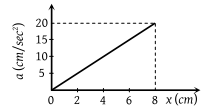(1) 8 × 10–2 joules

(2) 16 × 10–2 joules

(3) 4 × 10–4 joules

(4) 1.6 × 10–3 joules

Concept Questions :-

Work done by variable force
High Yielding Test Series + Question Bank - NEET 2020

Difficulty Level:

A toy car of mass 5 kg moves up a ramp under the influence of force F plotted against displacement x. The maximum height attained is given by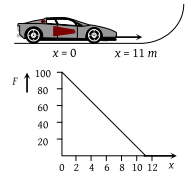(1) ymax = 20 m

(2) ymax = 15 m

(3) ymax = 11 m

(4) ymax = 5 m

Concept Questions :-

Gravitational Potential energy
High Yielding Test Series + Question Bank - NEET 2020

Difficulty Level:

The graph between the resistive force F acting on a body and the distance covered by the body is shown in the figure. The mass of the body is 25 kg and initial velocity is 2 m/s. When the distance covered by the body is 4m, its kinetic energy would be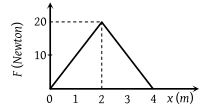(1) 50 J

(2) 40 J

(3) 20 J

(4) 10 J

Concept Questions :-

Work done by variable force
High Yielding Test Series + Question Bank - NEET 2020

Difficulty Level:

A particle of mass 0.1 kg is subjected to a force which varies with distance as shown in fig. If it starts its journey from rest at x = 0 , its velocity at x = 12 m is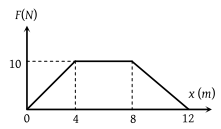(1) 0 m/s

(2) $20\sqrt{2}\text{\hspace{0.17em}}m/s$

(3) $20\sqrt{3}\text{\hspace{0.17em}}m/s$

(4) 40 m/s

Concept Questions :-

Work done by variable force